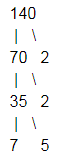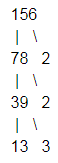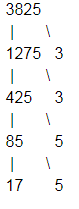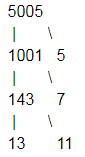Courses
Courses for Kids
Free study material
Free LIVE classes
MoreLIVE
Join Vedantu’s FREE Mastercalss

# Express each number as a product of its prime numbers:(i) 140(ii) 156(iii) 3825(iv) 5005(v) 7429Verified
270.3k+ views
Hint: First understand that the given numbers have to be divided by prime numbers which are also the factors of the numbers. Then convert the numbers into the product of its prime factors and write it as it is.

We are given some numbers and asked to express them as a product of their prime factors.
First we will understand what a prime factor is.
A prime factor is a prime number. In other words any of the prime numbers that will be multiplied to give the original number is a prime factor.
For example: $15=3\times 5$, here 3 and 5 are prime numbers and factors of 15.
We will find the prime factors by dividing the number by the prime number until its quotient is a prime number or 1.
In this question the numbers given are,
(i) 140 (ii) 156 (iii) 3825 (iv) 5005 (v) 7429
Let’s take 140,Now 140 can be expressed as $2\times 2\times 5\times 7$.
So, $140={{2}^{2}}\times 5\times 7$
Let’s take 156,Now 156 can be expressed as $2\times 2\times 3\times 13$.
So, $156={{2}^{2}}\times 3\times 13$.
Let’s take 3825.Now 3825 can be expressed as $3\times 3\times 5\times 5\times 17.$
So, $3825={{3}^{2}}\times {{5}^{2}}\times 17.$
Let’s take 5005,Now 5005 can be expressed as $5\times 7\times 11\times 13.$
So, $5005=5\times 7\times 11\times 13.$
Let’s take 7429,Now 7429 can be expressed as $17\times 19\times 23.$
So, $7429=17\times 19\times 23.$
\begin{align} & 140={{2}^{2}}\times 5\times 7 \\ & 156={{2}^{2}}\times 3\times 13 \\ & 3825={{3}^{2}}\times {{5}^{2}}\times 17 \\ & 5005=5\times 7\times 11\times 13 \\ & 7429=17\times 19\times 23 \\ \end{align}